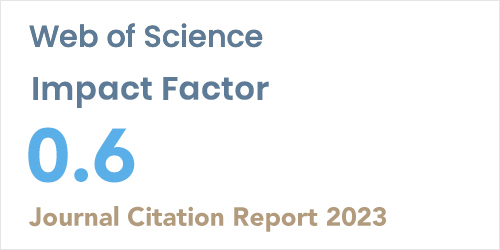ISSN: 1304-7191 | E-ISSN: 1304-7205
Volume: 41 Issue: 4 Year: 2023On Ev-Degree and Ve-Degree Based Topological Properties of the Sierpiński Gasket Fractal
1Faculty of Education, Van Yüzüncü Yıl University, VAN
Sigma J Eng Nat Sci 2019; 37(4): 1275-1280

#### Abstract

In chemistry, pharmacology, medicine and physics molecular graphs have been used to model molecular substances, networks and fractals. Topological indices have been derived from the molecular graphs of chemical compounds, networks and fractals. Topological indices are important tools to analyze the underlying topology of fractals. Many topological indices have been used to understand and to investigate mathematical properties of fractal models. The Sierpiński gasket fractal is important for the study of fractals. Some physical properties of these type fractals were investigated by some researchers. Also certain topological indices of the Sierpiński gasket fractal have been calculated recently. Ve-degree and Ev-degree concepts have been defined recently in graph theory. Ev-degree and Ve-degree topological indices have been defined by using their corresponding classical degree based topological indices. In this study we calculate ev-degree and ve-degree topological indices for the Sierpiński gasket fractal.